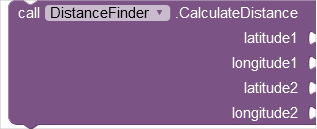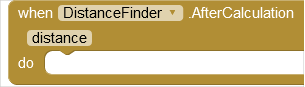DistanceFinder

This extension was created by Manan Sharma for calculating distance between two places. Thank You

💡 Current Version 1.0
📁 File Size 11.84 KB
📦 io.horizon.distancefinder.DistanceFinder
📅 Created On 2021-12-15

Method BlocksCalculateDistance - This block calculates the distance between two places
latitude1 input type number
longitude1 input type number
latitude2 input type number
longitude2 input type number

Event BlocksAfterCalculation - Event Triggered after calculating distance
distance output type number

Helper blocks

This extension was made on request of @Coding_Bot
and he has also tested the extension

This docs is generated from AIX Docs - Cttricks. Hitbutton if you liked this extension and feel free to comment below your reviews and suggestion.

Thank you

7 Likes

which formula do you use to calculate the distance?
the Haversine formula?

Taifun

1 Like

if a formula used (not web api), then maybe the AfterCaculated event is not needed. it can give a result directly.

2 Likes

Yes @Taifun I have used this formula only

Thank you
Horizon